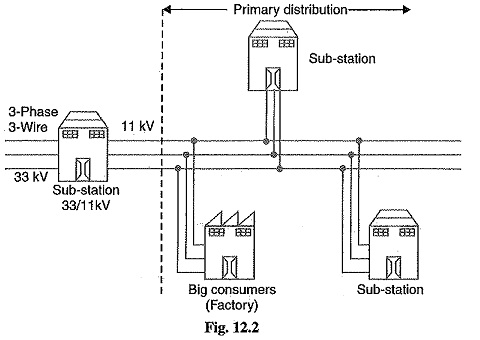## AC Distribution System:

AC Distribution Calculations : AC Distribution Calculations differ from those of d.c. distribution in the following respects : In case of d.c. system, the voltage drop is due to resistance alone. However, in a.c. system, the voltage drops are due to the combined effects of resistance, inductance and capacitance. In a d.c. system, additions and subtractions of currents or voltages are done arithmetically but in case of a.c. system, these operations are done vectorially. In an a.c. system, power factor (p.f.) has to be taken into account. Loads tapped off form the distributor are generally at different power factors. There are two ways of referring power factor viz It may be referred to supply or receiving end voltage which is regarded as the reference It may be referred to the voltage at the load point itself. There are several ways of solving AC Distribution Calculations. However, symbolic notation method has been found to be most convenient for this purpose. In this method, voltages, currents and imped­ances are expressed in complex notation and the calculations are made exactly as …